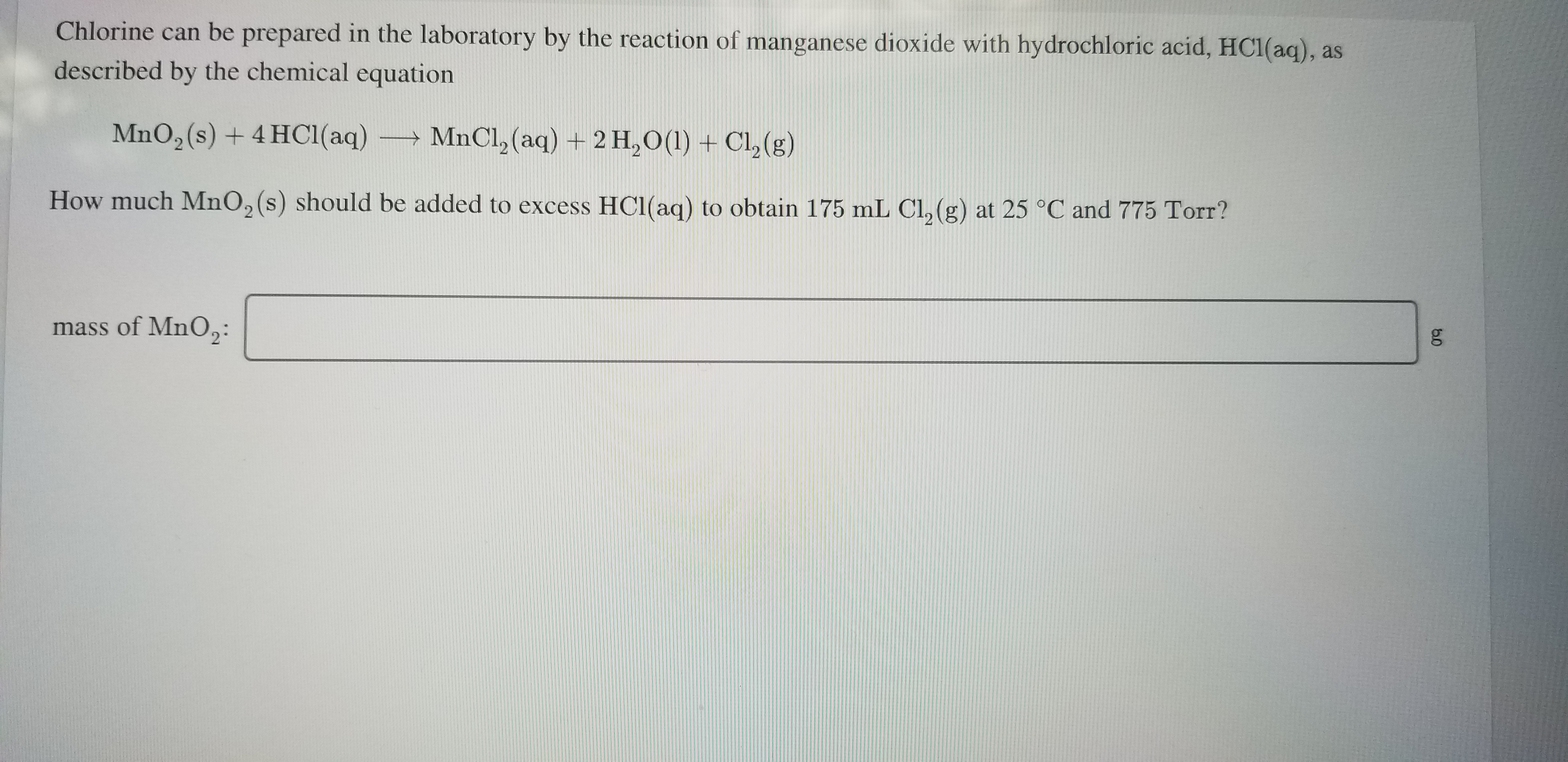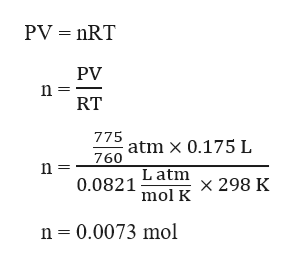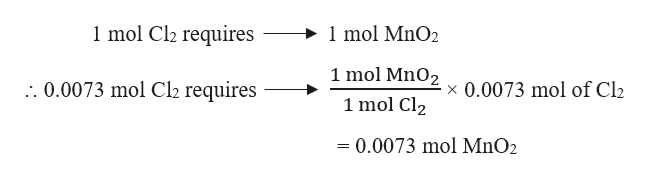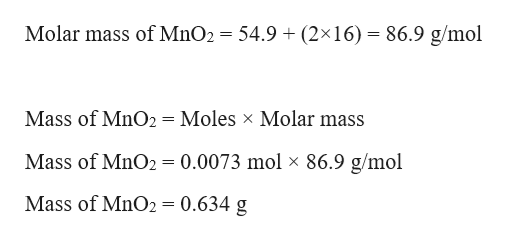# Chlorine can be prepared in the laboratory by the reaction of manganese dioxide with hydrochloric acid, HC1(aq), asdescribed by the chemical equationMnO,(s) + 4 HC1(aq)MnCl, (aq) + 2 H,O(1) + Cl,(g)How much MnO, (s) should be added to excess HC1(aq) to obtain 175 mL Cl, (g) at 25 °C and 775 Torr?mass of MnO,:

Question
1 viewshelp_outlineImage TranscriptioncloseChlorine can be prepared in the laboratory by the reaction of manganese dioxide with hydrochloric acid, HC1(aq), as described by the chemical equation MnO,(s) + 4 HC1(aq) MnCl, (aq) + 2 H,O(1) + Cl,(g) How much MnO, (s) should be added to excess HC1(aq) to obtain 175 mL Cl, (g) at 25 °C and 775 Torr? mass of MnO,: fullscreen
check_circle

Step 1

Given,

Volume of Cl2 gas, V = 175 mL = 0.175 L    (1 mL = 0.001 L)

Temperature, T = 25 °C = (25 + 273) K = 298 K

Pressure, P = 775 torr = 775/760 atm    (1 torr = 1/760 atm)

No. of moles (n) of Cl2 gas can be calculated using the ideal gas equation as:help_outlineImage TranscriptionclosePV = nRT PV n = RT 775 atm x 0.175 L 760 n = 0.0821 Latm x 298 K mol K n = 0.0073 mol fullscreen
Step 2

The balanced chemical reaction is given as:

MnO2 (s) + 4 HCl (aq) → MnCl2 (aq) + 2 H2O (l) + Cl2 (g)

From the given reaction, it is evident that :help_outlineImage Transcriptionclose1 mol Cl2 requires → 1 mol MnO2 1 mol MnO2 1 mol Cl2 .. 0.0073 mol Cl2 requires x 0.0073 mol of Cl2 = 0.0073 mol MnO2 fullscreen
Step 3

Mass of MnO2 required can be ...help_outlineImage TranscriptioncloseMolar mass of MnO2 = 54.9 + (2×16) = 86.9 g/mol Mass of MnO2 = Moles x Molar mass %3D Mass of MnO2 = 0.0073 mol × 86.9 g/mol Mass of MnO2 = 0.634 g fullscreen

### Want to see the full answer?

See Solution

#### Want to see this answer and more?

Solutions are written by subject experts who are available 24/7. Questions are typically answered within 1 hour.*

See Solution
*Response times may vary by subject and question.
Tagged in

### General Chemistry